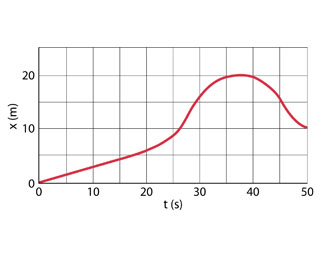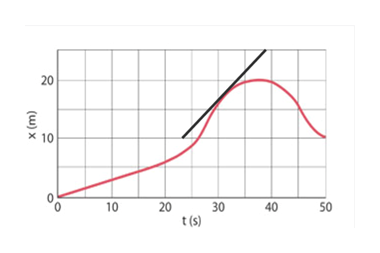2010304050t (s)x (m)20Of

Question

The position of a rabbit along a straight tunnel as a function of time is plotted in the figure.

What is its instantaneous velocity at t=10.0s?

What is its instantaneous velocity at t=30.0s?

What is its average velocity between t=0 and t=5.0s?

What is its average velocity between t=25.0s and t=30.0s?

What is its average velocity between t=40.0s and t=50.0s?help_outlineImage Transcriptionclose20 10 30 40 50 t (s) x (m) 20 Of fullscreen
Step 1

Instantaneous velocity is the velocity of an object in motion at a specific point in time.

Instantaneous speed is the slope of displacement curve.

For t=10s

Step 2

Draw a tangent line at t=30s and find its slope to get the velocityhelp_outlineImage Transcriptionclose20 x10 10 40 30 t(s) 50 20 (w)X fullscreen
Step 3

The tangent end co-ordinates a...

Want to see the full answer?

See Solution

Want to see this answer and more?

Our solutions are written by experts, many with advanced degrees, and available 24/7

See Solution
Tagged in

Physics